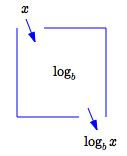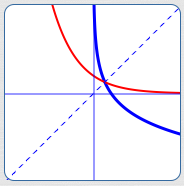﻿ Logarithm Summary: Properties, Formulas, Laws

# LOGARITHM SUMMARY: PROPERTIES, FORMULAS, LAWS

by Dr. Carol JVF Burns (website creator)
Follow along with the highlighted text while you listen!
• PRACTICE (online exercises and printable worksheets)

Logarithms and logarithmic functions have been thoroughly covered in:

This section provides an in-a-nutshell, at-a-glance summary of key results.

 What is $\,\log_b x\,$? Logarithms are exponents! The number  ‘$\,\log_b x\,$’  is the power (the exponent) that $\,b\,$ must be raised to, in order to get $\,x\,.$ $$\cssId{s7}{\log_b x = y\ \ \iff\ \ b^y = x}$$ Read  ‘$\,\log_b x\,$’  as  ‘log base $\,b\,$ of $\,x\,$’ . The equation  ‘$\,\log_b x = y\,$’  is called the logarithmic form of the equation. The equation  ‘$\,b^y = x\,$’  is called the exponential form of the equation. $\log_2 8 = 3\,$ since $\,2^3 = 8\,$ $\log_7 1 = 0\,$ since $\,7^0 = 1\,$ $\log_3 \frac 13 = -1\,$ since $\,3^{-1} = \frac 13\,$ Allowable Bases for Logs In the expression  ‘$\,\log_b x\,$’ , the number $\,b\,$ is called the base of the logarithm. The number $\,b\,$ must be positive and not equal to $\,1\,$:   $\,b > 0\,$ and $\,b\ne 1\,$allowable bases for logarithms: $b > 0\,,$ $\,b\ne 1$ Two Special Logarithms The function  ‘$\,\log_{10}\,$’  (log base $\,10\,$) is called the common logarithm. It is often abbreviated as just  ‘$\,\log\,$’  (with no indicated base). The function  ‘$\,\log_{\text{e}}\,$’  (log base $\,\text{e}\,$) is called the natural logarithm. It is often abbreviated as  ‘$\,\ln$’  (with no indicated base). Caution: Some disciplines use  ‘$\,\log\,$’  to mean the natural logarithm. Always check notation. $\log x$ the common log of $\,x$ $\ln x$ the natural log of $\,x$ Allowable Inputs for Logs In the expression  ‘$\,\log_b x\,$’ , the number $\,x\,$ (the input) must be positive: $\,x > 0\,$allowable inputs for logarithms: $x > 0$ Function View of Logs The number ‘$\log_b x\,$’ is the output from the function ‘$\,\log_b\,$’ when the input is ‘$\,x\,$’. The domain of the function $\,\log_b\,$ is the set of all positive real numbers:   $\,\text{dom}(\log_b) = (0,\infty)$ The range of the function $\,\log_b\,$ is the set of all real numbers: $\,\text{ran}(\log_b) = \Bbb R$function view of logarithms Laws of Logarithms Let $\,b\gt 0\,,$ $\,b\ne 1\,,$ $\,x\gt 0\,,$ and $\,y\gt 0\,.$ $\log_b\,xy = \log_b x + \log_b y$ The log of a product is the sum of the logs. $\displaystyle \log_b\frac{x}{y} = \log_b x - \log_b y$ The log of a quotient is the difference of the logs. For this final property, $\,y\,$ can be any real number: $\log_b\,x^y = y\,\log_b x$ You can bring exponents down. See Properties of Logarithms for a typical proof of these laws. Change of Base Formula for Logarithms Let $\,a\,$ and $\,b\,$ be positive numbers that are not equal to $\,1\,,$ and let $\,x\gt 0\,.$ Then, $$\cssId{s54}{\log_b\,x = \frac{\log_a\,x}{\log_a\,b}}$$ In words: You can change from any base $\,b\,$ to any base $\,a\,$; the ‘adjustment’ is that you must divide by the log to the new base ($\,a\,$) of the old base ($\,b\,$). See Change of Base Formula for Logarithms for a derivation of this formula. The equation $$\,\cssId{s60}{\log_b x = \left(\frac 1{\log_a b}\right)(\log_a x)}\,$$ shows that any log curve is just a vertical scaling of any other log curve! Logarithm functions are one-to-one Since logarithms are functions: $x = y \ \ \Rightarrow\ \ \log_b x = \log_b y$ When inputs are the same, outputs are the same. Since logarithms are one-to-one: $\log_b x = \log_b y\ \ \Rightarrow\ \ x = y$ When outputs are the same, inputs are the same. Thus, for all $\,b > 0\,,$ $\,b\ne 1\,,$ $\,x > 0\,$ and $\,y > 0\,$: $$\cssId{s70}{x = y\ \ \iff\ \ \log_b x = \log_b y}$$ Inverse Properties Logarithmic functions are one-to-one, hence have inverses. The inverse of the logarithmic function with base $\,b\,$ is the exponential function with base $\,b\,.$ For $\,b > 0\,,$ $\,b\ne 1\,,$ and all real numbers $\,x\,$: $$\cssId{s76}{\log_b b^x = x}$$ For $\,b > 0\,,$ $\,b \ne 1\,,$ and $\,x > 0\,$: $$\cssId{s78}{b^{\log_b x} = x}$$ Special Points For $\,b > 0\,$ and $\,b\ne 1\,$: $\log_b b = 1\,$   (since $\,b^1 = b\,$) Equivalently, the point $\,(b,1)\,$ lies on the graph of $\,y = \log_b x\,.$ $\log_b 1 = 0\,$   (since $\,b^0 = 1\,$) Equivalently, the point $\,(1,0)\,$ lies on the graph of $\,y = \log_b x\,.$

 Graphs of Logarithmic Functions Properties shared by all logarithmic graphs, $\,y = \log_b x\,$: vertical asymptote: $\,x = 0$ pass both horizontal and vertical line tests contain the point $\,(1,0)\,$ contain the point $\,(b,1)\,$ domain is the set of all positive real numbers range is the set of all real numbers the inverse of  $\,y = \log_b x\,$  is  $\,y = b^x\,$ the graph of the inverse is shown in red on the graphs at right: the inverse is the reflection of the logarithmic graph about the line $\,y = x\,$ For $\,b > 1\,$: $y = \log_b x\,$ is an increasing function: $$\cssId{s98}{x < y\ \ \iff\ \ \log_b x < \log_b y}$$ right-hand end behavior: as $\,x\rightarrow\infty\,,$ $\,y\rightarrow\infty$ $\,x = 0\,$ is a vertical asymptote: as $\,x\rightarrow 0^{+}\,,$ $\,y\rightarrow -\infty\,$$\,b > 1\,$: blue curve: $\,y = \log_b x\,$ red curve: inverse $\,y = b^x\,$ dashed line: $\,y = x\,$ For $\,0 < b < 1\,$: $y = \log_b x\,$ is a decreasing function: $$\cssId{s109}{x < y\ \ \iff\ \ \log_b x > \log_b y}$$ right-hand end behavior: as $\,x\rightarrow\infty\,,$ $\,y\rightarrow -\infty$ $\,x = 0\,$ is a vertical asymptote: as $\,x\rightarrow 0^{+}\,,$ $\,y\rightarrow \infty\,$$\,0 < b < 1\,$: blue curve: $\,y = \log_b x\,$ red curve: inverse $\,y = b^x\,$ dashed line: $\,y = x\,$
Master the ideas from this section